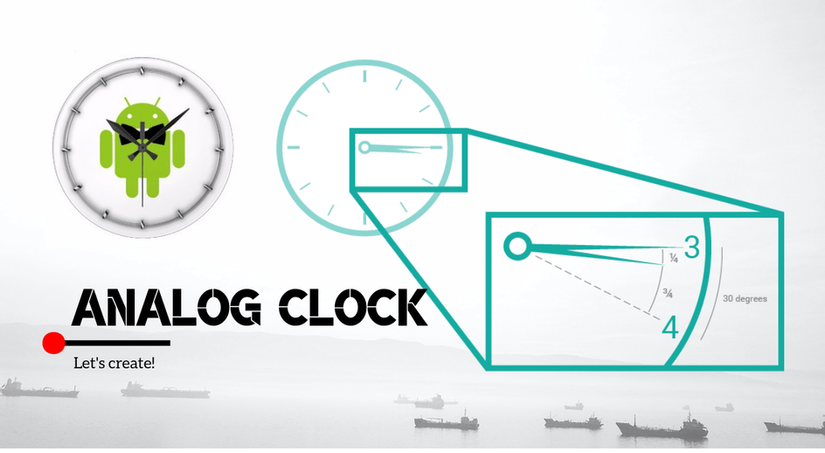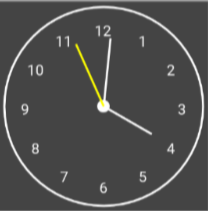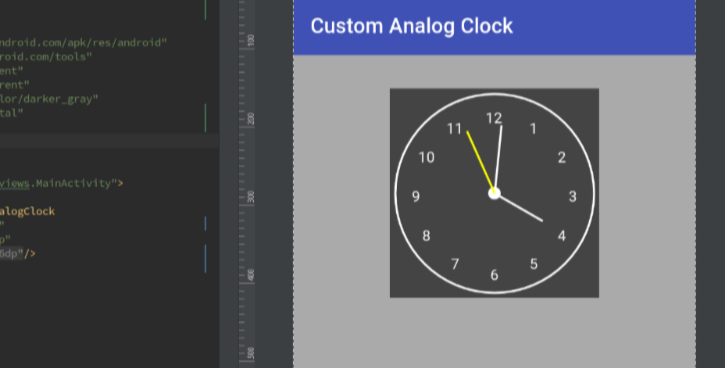+5

# Simple way to create a custom Analog clock in AndroidAndroid is a very popular operating system developed by Google which is a widely-used open-source project to become a huge platform of customized apps. Nowadays, many app developers as well as investors like to customize the UI portion of their apps. Going with the flow, I am going to describe about creating a custom analog clock in a simple way!

## Step - 1: Create a custom view class in Java

First of all, let's create a blank android project where you can add a custom View class namely `CustomAnalogClock.java` as following:

``````public class CustomAnalogClock extends View {

public CustomAnalogClock(Context context, AttributeSet attrs) {
super(context, attrs);
}

public CustomAnalogClock(Context context, AttributeSet attrs, int defStyleAttr) {
super(context, attrs, defStyleAttr);
}

@Override
protected void onDraw(Canvas canvas) {
// TODO: Implementation of the analog-clock UI in runtime goes here.
}
}
``````

## Step - 2: Design the clock UI

Suppose, we are going to create a round analog clock by extending `View` class which will be looked like the following picture.So, basically we will need:

• Round border (you can use rectangle or any other shape).
• 03 clock hands denoting hour, minute and second (I am denoting the "second" clock-hand using yellow color & the height of "hour" clock-hand is smaller comparetively to other 02 hands).
• Center point by which the 03 clock hands will be rotated.
• Hour points (e.g. 12 points/locations for 1h, 2h, 3h, .... ,12h), the hour points can be donoted by dots or numeric numbers.

## Step - 3: Declaring necessesary variables

We need some variables to process the UI in runtime using the `onDraw()` method as declaring below.

``````public class CustomAnalogClock extends View {

/** height, width of the clock's view */
private int mHeight, mWidth = 0;

/** numeric numbers to denote the hours */
private int[] mClockHours = {1, 2, 3, 4, 5, 6, 7, 8, 9, 10, 11, 12};

/** spacing and padding of the clock-hands around the clock round */
private int mNumeralSpacing = 0;

/** truncation of the heights of the clock-hands,
hour clock-hand will be smaller comparetively to others */
private int mHandTruncation, mHourHandTruncation = 0;

/** others attributes to calculate the locations of hour-points */
private Paint mPaint;
private Rect mRect = new Rect();
private boolean isInit;  // it will be true once the clock will be initialized.

public CustomAnalogClock(Context context, AttributeSet attrs) {
super(context, attrs);
}

...
}
``````

## Step - 4: Implement the UI & logic using the onDraw() method

Now, it's time to create a runtime UI logic for the analog-clock. Since, a `View` can draw only a single image-like representation, so we need to utilize the `onDraw()` method to redraw the every representation when time changes. And, everytime we need to update the UI of the hand-clocks as the second, minute and hour change. That's the way works as following:

``````public class CustomAnalogClock extends View {
....
@Override
protected void onDraw(Canvas canvas) {
/** initialize necessary values */
if (!isInit) {
mPaint = new Paint();
mHeight = getHeight();
mWidth = getWidth();
mPadding = mNumeralSpacing + 50;  // spacing from the circle border
int minAttr = Math.min(mHeight, mWidth);

// for maintaining different heights among the clock-hands
mHandTruncation = minAttr / 20;
mHourHandTruncation = minAttr / 17;

isInit = true;  // set true once initialized
}

/** draw the canvas-color */
canvas.drawColor(Color.DKGRAY);
}
}
``````

Now, we can create a border and a clock-center as following:

``````public class CustomAnalogClock extends View {

@Override
protected void onDraw(Canvas canvas) {

/** circle border */
mPaint.reset();
mPaint.setColor(Color.WHITE);
mPaint.setStyle(Paint.Style.STROKE);
mPaint.setStrokeWidth(4);
mPaint.setAntiAlias(true);
canvas.drawCircle(mWidth / 2, mHeight / 2, mRadius + mPadding - 10, mPaint);

/** clock-center */
mPaint.setStyle(Paint.Style.FILL);
canvas.drawCircle(mWidth / 2, mHeight / 2, 12, mPaint);  // the 03 clock hands will be rotated from this center point.
}
}
``````

After basic setup, it's time to create the vital part of the UI. In this stage, we need to make a round border of the 12 points denoting the hours as following:

``````public class CustomAnalogClock extends View {

@Override
protected void onDraw(Canvas canvas) {

/** border of hours */

int fontSize = (int) TypedValue.applyDimension(TypedValue.COMPLEX_UNIT_SP, 14, getResources().getDisplayMetrics());
mPaint.setTextSize(fontSize);  // set font size (optional)

for (int hour : mClockHours) {
String tmp = String.valueOf(hour);
mPaint.getTextBounds(tmp, 0, tmp.length(), mRect);  // for circle-wise bounding

// find the circle-wise (x, y) position as mathematical rule
double angle = Math.PI / 6 * (hour - 3);
int x = (int) (mWidth / 2 + Math.cos(angle) * mRadius - mRect.width() / 2);
int y = (int) (mHeight / 2 + Math.sin(angle) * mRadius + mRect.height() / 2);

canvas.drawText(String.valueOf(hour), x, y, mPaint);  // you can draw dots to denote hours as alternative
}
}
}
``````

Finally, we need to write the runtime logic for the clock-hands to rotate as following:

``````public class CustomAnalogClock extends View {

@Override
protected void onDraw(Canvas canvas) {

/** draw clock hands to represent the every single time */

Calendar calendar = Calendar.getInstance();
float hour = calendar.get(Calendar.HOUR_OF_DAY);
hour = hour > 12 ? hour - 12 : hour;

drawHandLine(canvas, (hour + calendar.get(Calendar.MINUTE) / 60) * 5f, true, false); // draw hours
drawHandLine(canvas, calendar.get(Calendar.MINUTE), false, false); // draw minutes
drawHandLine(canvas, calendar.get(Calendar.SECOND), false, true); // draw seconds

/** invalidate the appearance for next representation of time  */
postInvalidateDelayed(500);
invalidate();
}

private void drawHandLine(Canvas canvas, double moment, boolean isHour, boolean isSecond) {
double angle = Math.PI * moment / 30 - Math.PI / 2;
if (isSecond) mPaint.setColor(Color.YELLOW);
canvas.drawLine(mWidth / 2, mHeight / 2, (float) (mWidth / 2 + Math.cos(angle) * handRadius), (float) (mHeight / 2 + Math.sin(angle) * handRadius), mPaint);
}
}
``````

After writing all the above code-snaps, the analog-clock view class is ready to test as the following snap: